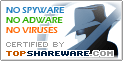# MatBasic 1.2

SNAPSHOT

The language of mathematical computing

Windows AllPlatform :
\$0Price :
3.4 MBFile Size :
ScreenshotScreenshot :Popularity :
User Reviews :MatBasic is a calculating, programming and debugging environment using special high-level programming language designed for solving mathematical problems. The MatBasic supports both the text and the graphical data visualization.

MatBasic is fast language interpreter and its environment application field is wide: from solving the school problem to executing different engineering and mathematical computations. The MatBasic programming language combines; simplicity of BASIC language, flexibility of high-level languages such as C or Pascal and at the same time turns up to be a powerful calculation tool. By means of a special operating mode, Matbasic it is possible to use as the powerful calculator. Also the MatBasic can be used for educational purpose as a matter of studying the bases of programming and raising algorithmization skills.

Matbasic is provide following calculation tools:
- Base calculation tools;
- Full complex arithmetic's;
- Linear algebra and operations. Solving systems of linear equations, eigenvalue problems, and singular value problems. Matrix factorizations (LU, Cholesky, QR, SVD, Schur);
- Polynomial problems. Roots of the polynomial. Polynomials multiplication, division and other functions;
- Numerically evaluate integral of function;
- Solve non-linear system of equations;
- Find zero of a function of one variable (solve non-linear equation);
- Minimize a function of one and more variables;
- Solve initial value problems for ordinary differential equations (stiff and non-stiff);
and others.

MATBASIC REVIEWS